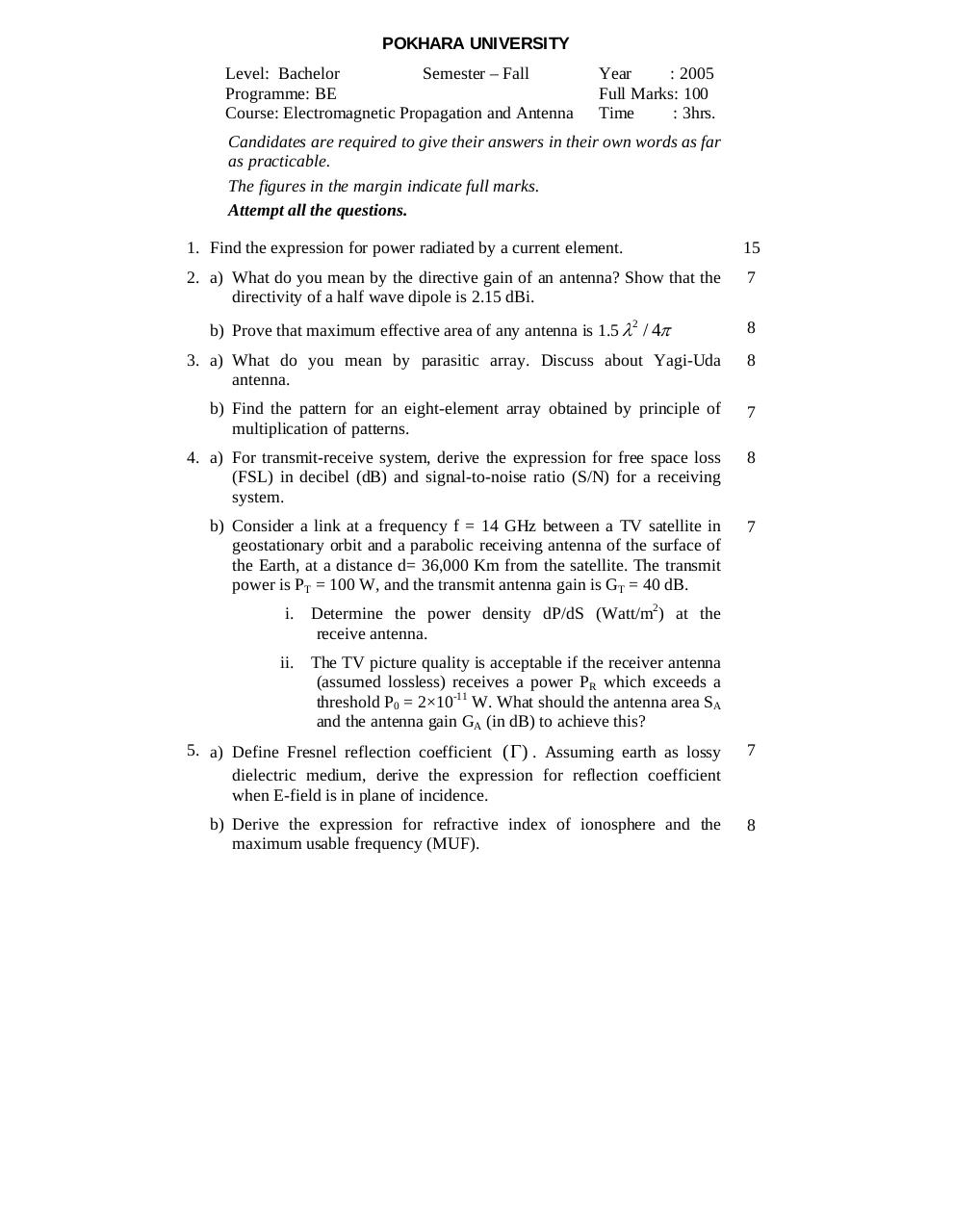# Antenna.pdfPage 123132

#### Text preview

POKHARA UNIVERSITY
Level: Bachelor
Semester – Fall
Programme: BE
Course: Electromagnetic Propagation and Antenna

Year
: 2005
Full Marks: 100
Time
: 3hrs.

Candidates are required to give their answers in their own words as far
as practicable.
The figures in the margin indicate full marks.
Attempt all the questions.
1. Find the expression for power radiated by a current element.

15

2. a) What do you mean by the directive gain of an antenna? Show that the
directivity of a half wave dipole is 2.15 dBi.

7

b) Prove that maximum effective area of any antenna is 1.5 λ2 / 4π

8

3. a) What do you mean by parasitic array. Discuss about Yagi-Uda
antenna.

8

b) Find the pattern for an eight-element array obtained by principle of
multiplication of patterns.

7

(FSL) in decibel (dB) and signal-to-noise ratio (S/N) for a receiving
system.

8

b) Consider a link at a frequency f = 14 GHz between a TV satellite in
geostationary orbit and a parabolic receiving antenna of the surface of
the Earth, at a distance d= 36,000 Km from the satellite. The transmit
power is PT = 100 W, and the transmit antenna gain is GT = 40 dB.

7

i. Determine the power density dP/dS (Watt/m2) at the
ii. The TV picture quality is acceptable if the receiver antenna
(assumed lossless) receives a power PR which exceeds a
threshold P0 = 2×10-11 W. What should the antenna area SA
and the antenna gain GA (in dB) to achieve this?
5. a) Define Fresnel reflection coefficient ( Γ) . Assuming earth as lossy
dielectric medium, derive the expression for reflection coefficient
when E-field is in plane of incidence.

7

b) Derive the expression for refractive index of ionosphere and the
maximum usable frequency (MUF).

8